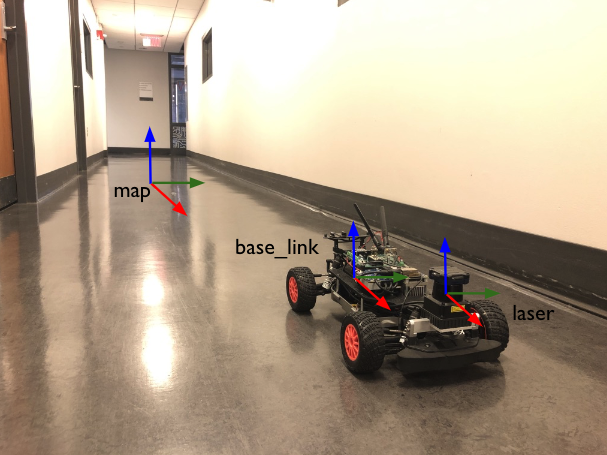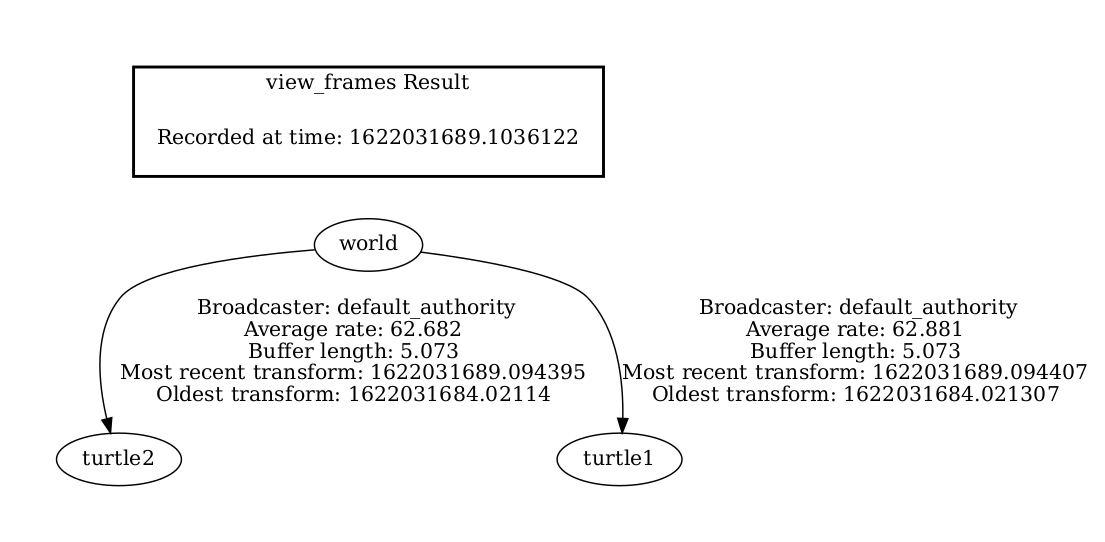Rigid-Body Transformations

# tf2 §

This is the implementation from ROS of Rigid-Body Transformations. I think that while I don’t have 100% theoretical background about transformations, I should be familiar with the practical side of things.

This is an aha moment: The way that a car can locate itself is by publishing to the tf2 tree.

• you have the odom topic that tells you how far the car is
• you have the odom frame that tells you the distance between map and base_linkThere are two types of transformations:

1. Static Transformations (ex: between laser and base_link), 1-time thing, never changes because they are fixed to each other
2. Dynamic Transformations (ex: between laser and map). As the car moves, the values change
def make_transforms(self, transformation):
t = TransformStamped()

t.child_frame_id = transformation

t.transform.translation.x = float(transformation)
t.transform.translation.y = float(transformation)
t.transform.translation.z = float(transformation)
quat = quaternion_from_euler(
float(transformation), float(transformation), float(transformation))
t.transform.rotation.x = quat
t.transform.rotation.y = quat
t.transform.rotation.z = quat
t.transform.rotation.w = quat

self.tf_static_broadcaster.sendTransform(t)

In the above example,

• header.frame_id is the source frame
• child_frame_id field is the frame ID of the coordinate frame that is being transformed into (i.e.target frame)

Resources

### Publish a static transform from terminal §

ros2 run tf2_ros static_transform_publisher 0 0 0 0 0 0 base_link laser


### Visualizing the TF Tree §

To see the current transforms being published (on Humble and up)

ros2 run tf2_tools view_frames

If you are on ROS Foxy, run

ros2 run tf2_tools view_frames.pyThis sames it to frames.pdf, and you can open it with a PDF viewer.

Also see rqt_graph to generate the computation graph with all the ROS2 nodes.

### Static Transformations §

I’ve added these before in a launch file, by running something like:

Node(package='tf2_ros', executable='static_transform_publisher',
arguments=['-0.012', '0', '0.144', '0', '0', '0', 'base_footprint', 'laser'])

### Dynamic Transformations §

#### Publish a Transform (Broadcaster) §

I have never done this. The particle filter code automatically does this.

Python, code here

self.tf_broadcaster.sendTransform(t)

C++, code here

tf_broadcaster_->sendTransform(t);

#### Lookup a transform (Listener) §

In the Pure Pursuit code for F1TENTH, we do this.

Python, code here

try:
t = self.tf_buffer.lookup_transform(to_frame_rel,from_frame_rel, rclpy.time.Time())
except TransformException as ex:
self.get_logger().info(
f'Could not transform {to_frame_rel} to {from_frame_rel}: {ex}')
return

C++, code here

try {
t = tf_buffer_->lookupTransform(toFrameRel, fromFrameRel, tf2::TimePointZero);
} catch (const tf2::TransformException & ex) {
RCLCPP_INFO(this->get_logger(), "Could not transform %s to %s: %s", toFrameRel.c_str(), fromFrameRel.c_str(), ex.what());
return;
}